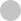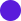#### Progress

0%#### Basic Data Structures#### Overview

•Vanilla Binary Search
•Finding Boundary

#### Sorted Array

•First Element Not Smaller Than Target 🔒
•First Occurrence
•Square Root 🔒

#### Implicitly Sorted Array#### Introduction

•Recursion Review
•DFS Fundamentals

#### Combinatorial Search

•DFS with States
•Backtracking Fundamentals
•Permutations
•Letter Combinations of Phone Number 🔒

#### Memoization

•Memoization Intro 🔒
•Word Break 🔒
•Decode Ways 🔒#### Introduction

•BFS Fundamentals

#### BFS on Tree#### Introduction

•Graph Fundamentals
•BFS on Graphs 🔒
•DFS on Graph 🔒
•BFS or DFS 🔒
•Matrix as Graph 🔒

#### BFS

•Shortest Path 🔒
•Word Ladder 🔒

#### Matrix as Graph

•Flood Fill 🔒
•Number of Islands 🔒
•Knight Minimum Moves 🔒

#### Directed Graph

•Course Schedule 🔒#### Same Direction

•Remove Duplicates 🔒
•Middle of Linked List 🔒
•Move Zeros 🔒

#### Opposite Direction

•Two Sum Sorted 🔒
•Valid Palindrome 🔒
•Trapping Rain Water 🔒

#### Prefix Sum

•Subarray Sum 🔒
•Subarray Sum Divisible by K 🔒#### Introduction

•Heap Fundamentals

#### Top K

•K Closest points 🔒
•Merge K Sorted Lists 🔒#### Binary Search#### Sequence

•House Robber 🔒
•Coin Change 🔒

#### Grid

•Number of Robot Paths 🔒
•Minimal Path Sum 🔒
•Maximal Square 🔒

#### Two Sequences

•Edit Distance 🔒
•Longest Common Subsequence 🔒

#### Game Theory

•Divisor Game 🔒#### Monotonic Stack

•Sliding Window Maximum

#### Data Structure Design

•LRU Cache 🔒# Find Element in Sorted Array with Duplicates

Given a sorted array of integers and a target integer, find the first occurrence of the target and return its index. Return -1 if the target is not in the array.

Input:

``````arr = [1, 3, 3, 3, 3, 6, 10, 10, 10, 100]
target = 3``````

Output:

``1``

Explanation: First occurrence of 3 is at index 1.

## Explanation

The problem is equivalent to finding the boundary of elements < 3 and elements >= 3. Imagine we apply a filter of `arr[i] <= 3`, we would get:Now the problem is reduced to finding the first `true` element in a boolean array. And we already know how to do this from Find Boundary module.

## Implementation

 `1` `1` ``def find_first_occurrence(arr, target):`` `2` `-` `` # WRITE YOUR BRILLIANT CODE HERE`` `2` `+` `` l, r = 0, len(arr) - 1`` `3` `+` `` ans = -1`` `4` `+` `` while l <= r:`` `5` `+` `` mid = (l + r) // 2`` `6` `+` `` if arr[mid] == target:`` `7` `+` `` ans = mid`` `8` `+` `` r = mid - 1`` `9` `+` `` elif arr[mid] < target:`` `10` `+` `` l = mid + 1`` `11` `+` `` else:`` `12` `+` `` r = mid - 1`` `13` `+` `` return ans`` `14` `+` `3` `15` ``if __name__ == '__main__':`` `4` `16` `` arr = [int(x) for x in input().split()]`` `5` `17` `` target = int(input())`` `6` `18` `` print(find_first_occurrence(arr, target))``